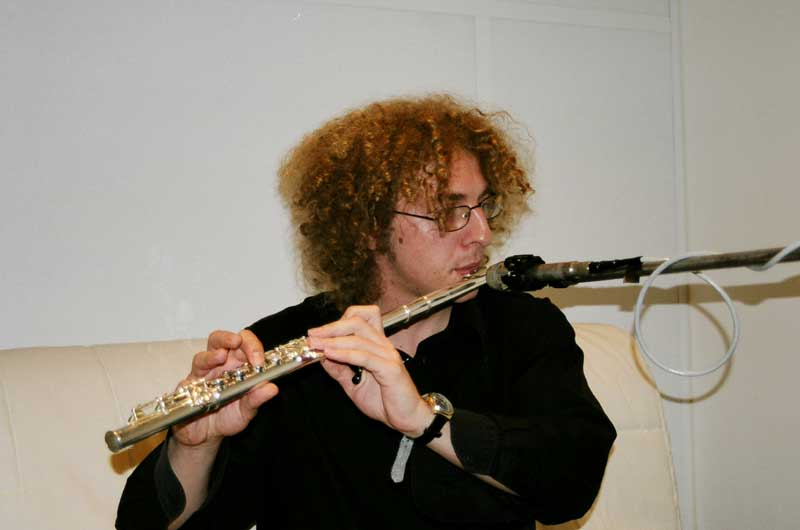Libor BartoDOMU VYZKUM PRO STUDENTY

ARCHIV 13/14 zimni semestr

[Zpet]

LINEARNI ALGEBRA A GEOMETRIE 1 (NMAG101)

UNIVERSAL ALGEBRA 1 (NMAG405)

Tutorial: Monday 10:40 - 12:10 K7 (instructor: Pavel Ruzicka)
Lecture: Thursday 14:00 - 15:30 K7

LECTURES

• 3.10. Introduction. Algebra (formalisms using types and signatures). Homomorfism, isomorphism.
• 10.10. Subuniverse, subalgebra. Product, power, subpower. Congruence, quotient.
• 17.10. Variety, V(K)=HSP(K). Homomorphisms and constructions. 3 Isomorphism Theorems, Correspondence theorem.
• 24.10. Lattice <-> lattice ordered set. Modular lattice. Thm: modular <=> no N5 <=> isomorphisms of intervals. Thm: modular => max. chains have the same length. Thms: congruences in groups permute => Con(group) is modular.
• 31.10. Distributive lattice. Thm: distributive <=> two majorities coincide <=> no N5, M3 <=> at most one complement. Thm: Con(lattice) is distributive. Thm: Distributive lattices = sublattice of P(X).
• 7.11. Complete lattice, complete sublattice, compact elements, algebraic lattice. Closure operator, algebraic closure operator. Correspondence: (algebraic) closure operators ... (algebraic) complete lattices.
• 14.11. Galois connection, induced closure operators, induced dual lattice isomorphism.
• 21.11. Direct decomposition, internal characterization. Subdirect decomposition, SI algebra, internal characterization, completely meet irreducible element, monolith. Thm: every algebra is (isomorphic to) a subdirect product of SI algebras. Crlr: Each variety is determined by SIs in it.
• 28.11. Term, evaluation, term operation, identity, satisfaction. Absolutely free algebra, free algebra for a class. Thm: Free algebra for K is in SP(K) and is equal to terms modulo identities true in K.
• 5.12. Galois connection between classes of algebras and sets of identities (over a countable infinite set X of variables). Thm (Birkhoff): Closed classes of algebras = varieties. Thm: Closed classes of identities = fully invariant congruences of the absolutely free algebra over X.
• 12.12. Clone, clone of an algebra, free algebras via clones. Galois connection between sets of operations and sets of relations - invariant relations, polymorphisms.
• 19.12. Thm: Closed sets of operations = clones. PP-definability, relational clone. Thm: Closed classes of relations = relational clones.
• 2.1. canceled
• 9.1. (not required for the exam) CSP

LITERATURE

• C. Bergmann: Universal Algebra, CRC Press, 2012. (Available in the library)
• S. Burris, H.P. Sankappanavar: A course in universal algebra, 1981. Online version (update 2012) here
• D. Stanovsky, Priklady z algebry, in Czech (exercises on general algebra are on pages 41-49)

SEMINAR K PROBLEMU CSP (NALG118)

ARCHIV